# 四象限里的正弦、余弦和正切

## 正弦、余弦和正切……但我们必须知道是哪一边！

θ 为角度，这些函数的计算方法是：

 正弦函数： sin(θ) = 对边 / 斜边 余弦函数： cos(θ) = 邻边 / 斜边 正切函数： tan(θ) = 对边 / 邻边

### 例子：35°的正弦是多少？用这个三角形（长度准确到一个小数位）： sin(35°) = 对边 / 斜边 = 2.8/4.9 = 0.57……

## 笛卡尔坐标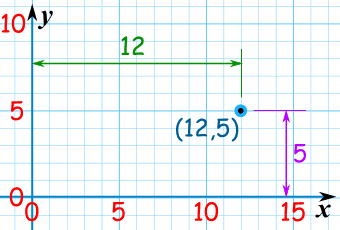(12,5) 是向右 12 单位，和向上 5 单位。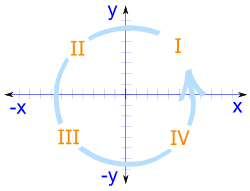## 四个象限

（以逆时针方向排序）

• 象限 I，x 和 y 两者皆为正数，
• 象限 II ，x 是负数（y 仍是正数），
• 象限 III x 和 y 两者皆为负数
• 象限 IV，x 再次是正数，而 y 是负数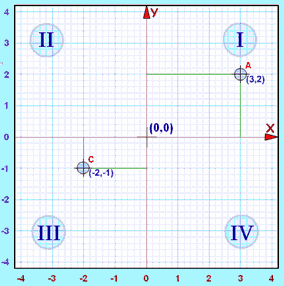（水平）
Y
（垂直）

I (3,2)
II
III (−2,−1)
IV

x 和 y 两者都是负数，所以点是在 "象限 III"

## 四个象限里的正弦、余弦和正切

### 例子：30°的正弦、余弦和正切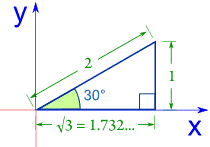正弦 sin(30°) = 1 / 2 = 0.5 余弦 cos(30°) = 1.732 / 2 = 0.866 正切 tan(30°) = 1 / 1.732 = 0.577

### 例子：150°的正弦、余弦和正切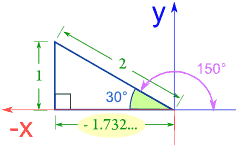正弦 sin(150°) = 1 / 2 = 0.5 余弦 cos(150°) = −1.732 / 2 = −0.866 正切 tan(150°) = 1 / −1.732 = −0.577

### 例子：210°的正弦、余弦和正切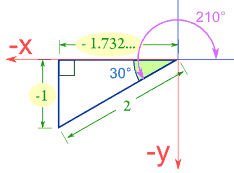正弦e sin(210°) = −1 / 2 = −0.5 余弦 cos(210°) = −1.732 / 2 = −0.866 正切 tan(210°) = −1 / −1.732 = 0.577

In 象限 IV，正弦和正切是负数：

### 例子：330°的正弦、余弦和正切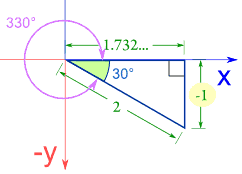正弦 sin(330°) = −1 / 2 = −0.5 余弦 cos(330°) = 1.732 / 2 = 0.866 正切 tan(330°) = −1 / 1.732 = −0.577

• 象限 I三个函数都是正数
• 象限 II只有正弦是正数
• 象限 III只有正切是正数
• 象限 IV只有余弦是正数你可以记着英语字母 ASTC，就是 (A)ll（全部）、(S)ine、(T)angent 和 (C)osine。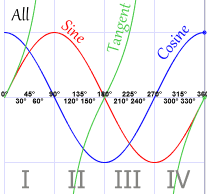这图也显示 "ASTC"。

## 两个值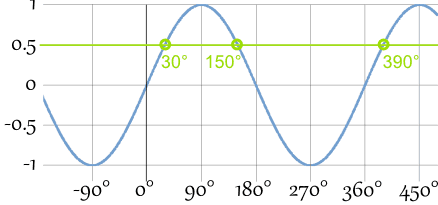（在头 360°里），有 两个角度的正弦是相同的！

……但你可以用以下的规则来求另一个答案：

 第一值 第二值 正弦 θ 180º − θ 余弦 θ 360º − θ 正切 θ θ − 180º